Next: Scattering of Identical Particles Up: Scattering Theory Previous: Resonant Scattering

# Elastic and Inelastic Scattering

According to the analysis of Section 10.5, for the case of a spherically symmetric scattering potential, the scattered wave is characterized by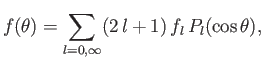(10.138)

where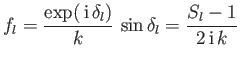(10.139)

is the amplitude of theth partial wave, whereas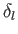is the associated phase-shift. Here,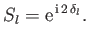(10.140)

Moreover, the fact that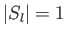ensures that the scattering is elastic (i.e., that the number of particles is conserved). Finally, according to the analysis of Section 10.6, the net elastic scattering cross-section can be written(10.141)

It turns out that many scattering experiments are characterized by the absorption of some of the incident particles. Such absorption may induce a change in the quantum state of the target, or, perhaps, the emergence of another particle. Note that scattering that does not conserve particle number is known as inelastic scattering. We can take inelastic scattering into account in our analysis by writing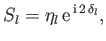(10.142)

where the real parameter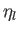is such that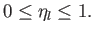(10.143)

It follows from Equation (10.140) that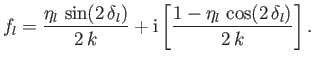(10.144)

Hence, according to Equation (10.142), the net elastic scattering cross-section becomes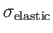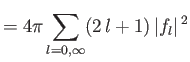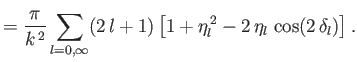(10.145)

The net inelastic scattering (i.e., absorption) cross-section follows from Equations (10.24) and (10.88):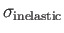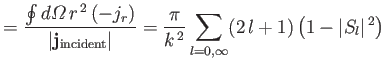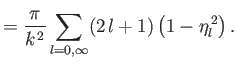(10.146)

Thus, the total cross-section is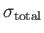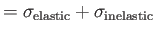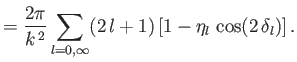(10.147)

Note, from Equations (10.139), (10.140), and (10.143) that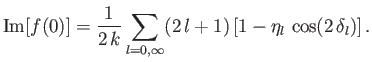(10.148)

In other words,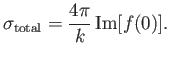(10.149)

Hence, we deduce that the optical theorem, described in Section 10.6, still applies in the presence of inelastic scattering.

If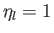then there is no absorption, and theth partial wave is scattered in a completely elastic manner. On the other hand, if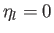then there is total absorption of theth partial wave. However, such absorption is necessarily accompanied by some degree of elastic scattering. In order to illustrate this important point, let us investigate the special case of scattering by a black sphere. Such a sphere has a well-defined edge of radius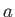, and is completely absorbing. Consider short-wavelength scattering characterized by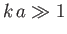. In this case, we expect all partial waves with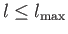, where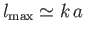, to be completely absorbed (because, by analogy with classical physics, the impact parameters of the associated particles are less than--see Section 10.8), and all other partial waves to suffer neither absorption nor scattering. In other words,for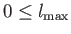, and,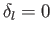for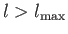. It follows from Equations (10.146) and (10.147) that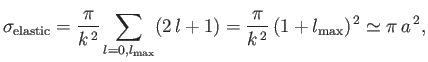(10.150)

and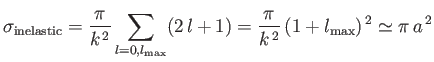(10.151)

. Thus, the total scattering cross-section is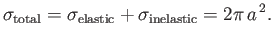(10.152)

This result seems a little strange, at first, because, by analogy with classical physics, we would not expect the total cross-section to exceed the cross-section presented by the sphere. Nor would we expect a totally absorbing sphere to give rise to any elastic scattering. In fact, this reasoning is incorrect. The absorbing sphere removes flux proportional to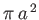from the incident wave, which leads to the formation of a shadow behind the sphere. However, a long way from the sphere, the shadow gets filled in. In other words, the shadow is not visible infinitely far downstream of the sphere. The only way in which this can occur is via the diffraction of some of the incident wave around the edges of the sphere. Actually, the amount of the incident wave that must be diffracted is the same amount as was removed from the wave by absorption. Thus, the scattered flux is also proportional to.

Consider low-energy scattering by a hard-sphere potential. As we saw in Section 10.8, this process is dominated by S-wave (i.e.,) scattering. Moreover, the phase-shift of the S-wave takes the form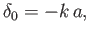(10.153)

where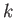is the wavenumber of the incident particles, andis the radius of the sphere. Note that the low-energy limit corresponds to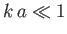. It follows that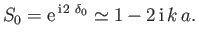(10.154)

We can generalize the previous analysis to take absorption into account by writing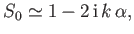(10.155)

where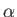is complex,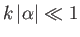, and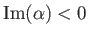. According to Equations (10.146) and (10.147),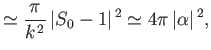(10.156)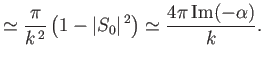(10.157)

We conclude that the low-energy elastic scattering cross-section is again independent of the incident particle velocity (which is proportional to), whereas the inelastic cross-section is inversely proportional to the particle velocity. Consequently, as the incident particle velocity decreases, inelastic scattering becomes more and more important in comparison with elastic scattering .Next: Scattering of Identical Particles Up: Scattering Theory Previous: Resonant Scattering
Richard Fitzpatrick 2016-01-22